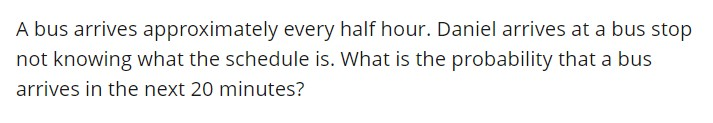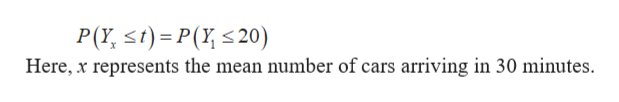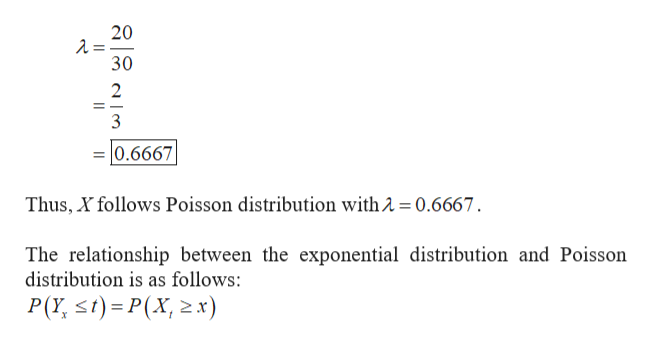A bus arrives approximately every half hour. Daniel arrives at a bus stopnot knowing what the schedule is. What is the probability that a busarrives in the next 20 minutes?

Question

Hi. How do you calculate this Poisson Distribution in the TI-83? Thanks.help_outlineImage TranscriptioncloseA bus arrives approximately every half hour. Daniel arrives at a bus stop not knowing what the schedule is. What is the probability that a bus arrives in the next 20 minutes? fullscreen
Step 1

Let ‘Y’ be the waiting time between two busses which follows exponential distribution with mean waiting time 30 minutes and arrival rate 1/30. That is, 1 bus in 30 minutes.

The probability that a bus arrives in the next 20 minutes is equal to waiting time less than or equal to 20 minutes. It can be written as,help_outlineImage TranscriptioncloseP( st) P(20) Here, x represents the mean number of cars arriving in 30 minutes. fullscreen
Step 2

Let X be the number of busses arriving in 20 minutes.

So, the avera...help_outlineImage Transcriptionclose20 -=Y 30 2 3 0.6667 Thus, X follows Poisson distribution with 2 0.6667 The relationship between the exponential distribution and Poisson distribution is as follows: P(Y st) P(X, x) fullscreen

Want to see the full answer?

See Solution

Want to see this answer and more?

Our solutions are written by experts, many with advanced degrees, and available 24/7

See Solution
Tagged in

Other